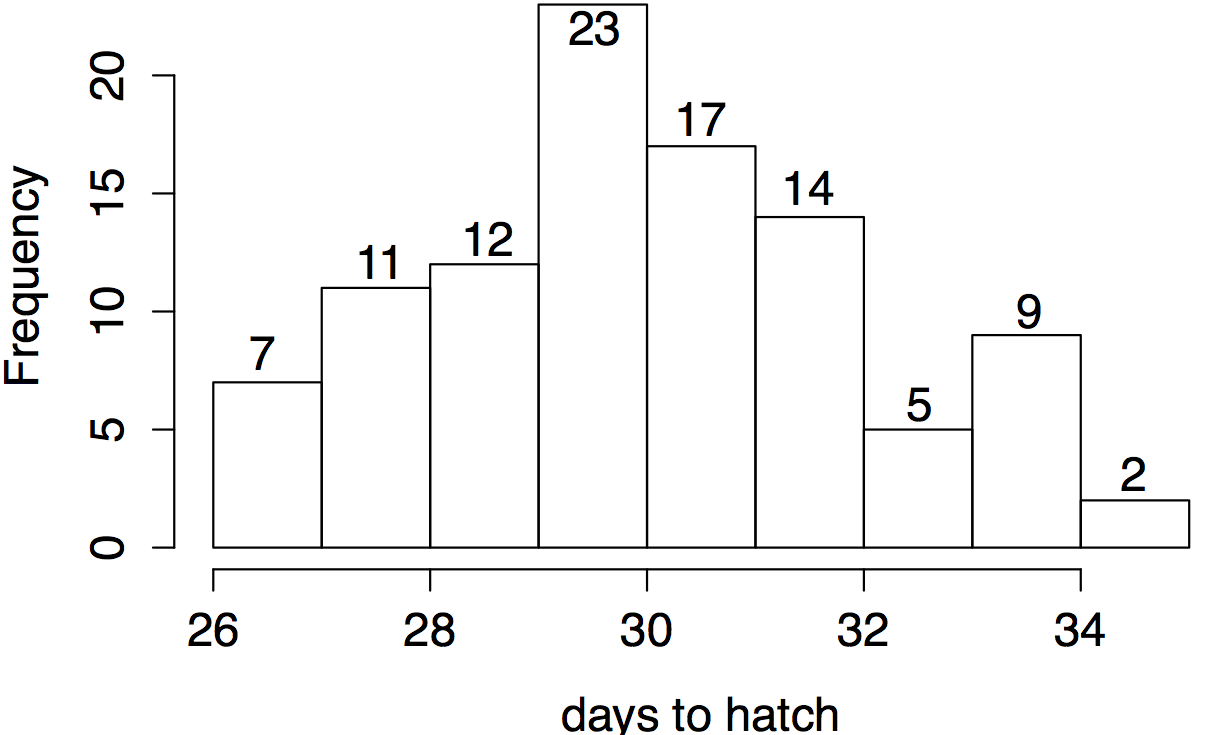# Exercise: time to hatch

22 Jun 2015

#Objective We are learning about random variables, distributions, expectation, and variance. This exercise is intended to help make the connections between population features (like probability distributions, random variables, and expectation) and concepts related to the sample, like realizations and the mean.

Meanwhile, I am emphasizing that the sample and the population are linked by probability in one direction, and inference in the other.

#Exercise

## Overview

An ornithologist observed a prairie clearing over the course of a summer. She found all the robin nests and noted when the birds laid their eggs (there were 100 eggs in total). She then measured how many days passed before each egg hatched.

Number of days until hatching are plotted in the histogram. Interpret the horizontal axis to say that birds who hatched between day 26 and 27 took 26 days to hatch (7 of them), those hatching between 27th and 28th days took 27 days to hatch (11 of them), etc.

## Part A

### 1### 2

$29.5 \;{\rm days}\; = 26 \frac{7}{100} \;+\; 27 \frac{11}{100} \;+\; 28 \frac{12}{100} \;+\; 29 \frac{23}{100} \;+\; 30 \frac{17}{100} \;+\; 31 \frac{14}{100} \;+\; 32 \frac{5}{100} \;+\; 33 \frac{9}{100} \;+\; 34 \frac{2}{100}$

%

## Part B

Now imagine that we write each egg’s time to hatch on a chip and put them all in an urn. We pick one chip at random, write down its number, then put it back. We repeat this to sample five numbers.

1. Numbers sampled: 32, 27, 27, 29, 30 days

## Part C

Fill in the blanks from the following word list. Some words may be used more than once, and there is more than one defensible answer for some blanks.

• mean
• standard deviation
• realization
• inference
• sample
• probability distribution
• expectation
• random variable
• population
1. Everything in part A relates to the ______
2. Everything in part B relates to the ______
3. A(2) is the ____ of the _____
4. A(3) is the ____ of the _____
5. B(2) is the _____ of the ______
6. B(3) is the _____ of the ______
7. In B(1), we have listed five ______s of a ______
8. The ________ of this random variable is plotted in A(1).
9. Estimating A(2) by B(2) or A(3) by B(3) is an example of _________

## Part D

To be done individually:

For a bonus participation point, write a few sentences that tie the whole exercise together, using as many words from the word list as possible.